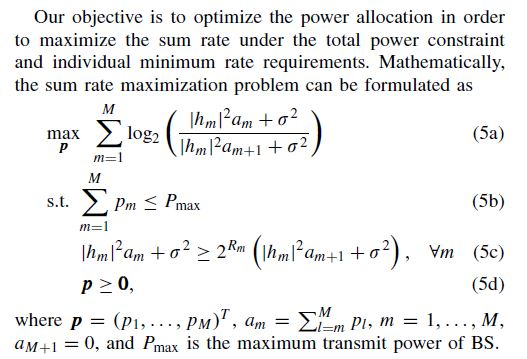# How to express log(x/y) in CVX?Hi everyone,
I had a problem reproducing a paper using CVX(such as the one illustrated above).
The problem (5) is convex and (5a) has been rigorously proved to be concave in the paper.
I want to know how can I express the objective function correctly in CVX?
Thanks.

In general, log(x/y) is neither convex nor concave. However, by virtue of the formula for a_m, there is a relation between the x and the y. This is sort of like log(x/(x+y)), which in general, is also neither convex nor concave. If this can be done, perhaps something similar to @Michal_Adamaszek 's technique in Can CVX solve this kind of function {x-log(1+0.01*x/(x+1))} can be used, although that problem only has a single variable, unlike the one you have presented.

Ir might help if you showed us the proof of concavity of the objective function. Is anything else being assumed than what is shown in the image?

I’ve spent a long time on CVX trying to solve this problem, but it hasn’t.
The rigorous proof of the above problems comes from a paper on IEEE Communications Letters. The proof process is too long for me to show it all on the Forum. However, the address of this paper on IEEE is https://ieeexplore.ieee.org/document/7890454 ,which I think may help me explain my problem better. What’s more, the proof of the concavity of the objective function was showed in the APPENDIX B of this paper.

If that problem can be formulated in CVX, your best best may be to contact the authors for assistance.

A google search showed the paper at

Btw given the h’s are sorted in increasing order then it is easy to show R in (23) in the above paper is concave I think. Note the Hessian of R is a diagonal matrix so you can analyze each diagonal element.

\begin{array}{rcl} \ln \left ( \frac{\alpha x + \sigma^2}{\beta x+ \sigma^2} \right ) & = & \ln(\frac{\alpha}{\beta} - \frac{\alpha}{\beta} \frac{\beta x+ \sigma^2}{\beta x+ \sigma^2} + \frac{\alpha x + \sigma^2}{\beta x+ \sigma^2}) \\ & = & \ln(\frac{\alpha}{\beta} - \frac{(\frac{\alpha}{\beta}-1) \sigma^2}{\beta x+ \sigma^2}) \end{array}

which is a concave function for \alpha \geq \beta > 0 and x \geq 0. Note x represents the a's and
\alpha and \beta are the h's in the paper. And I assume \sigma^2 > 0.

Now let

\ln \left ( \frac{\alpha x + \sigma^2}{\beta x+ \sigma^2} \right ) \geq \ln(s)

where

s \leq \frac{\alpha}{\beta} - \frac{(\frac{\alpha}{\beta}-1)\sigma^2}{\beta x+ \sigma^2}

This set is conic quadratic representable. So you just maximize ln(s) subject to this conic quadratic representable constraint.

Hence, to me it seems that (23) in the conic representable and therefore can be represented in CVX.

Finally I would express

\begin{array}{rcl} a_M & = & p_M \\ a_{M-1} & = & a_M + p_{M-1} \\ a_{M-2} & = & a_{M-1} + p_{M-2} \\ \end{array}

and so forth.

PS. I got a little help from @Michal_Adamaszek but all mistakes are mine.